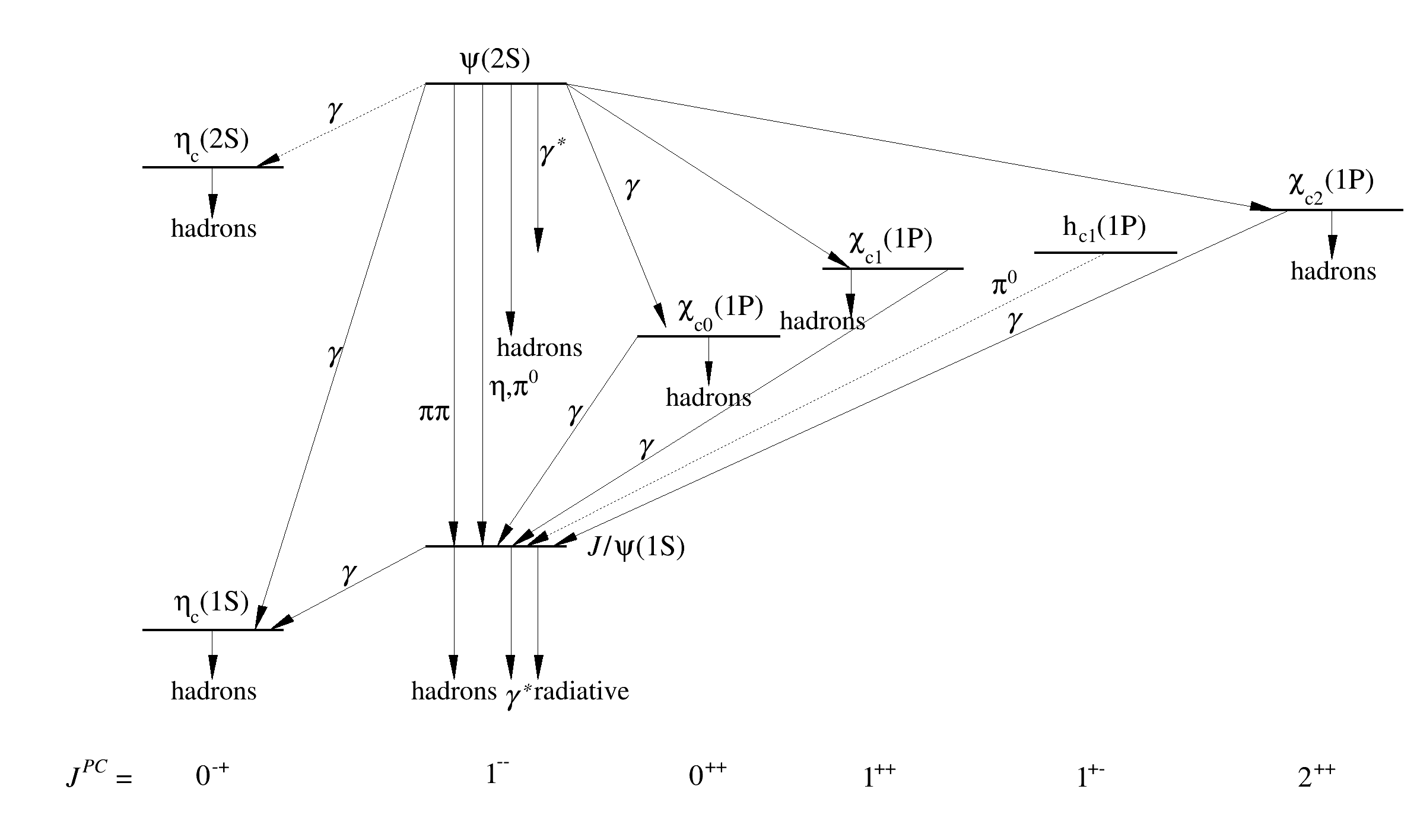ROOT   Reference Guidemass_spectrum.C File Reference

## Detailed DescriptionThis macro makes use of some basic graphics primitives such as line, arrow and text.

It has been written using the TCanvas ToolBar to produce a first draft and was then modified for fine adjustments. Note also the use of C functions. They allow to simplify the macro reading and editing by avoiding code repetition or defining some graphics attributes in one single place. This technique to generate drawings may appear not very user friendly compare to all the "wysiwyg" graphics editors available. In some cases it can be more powerful than a GUI interface because it allows to generate very precise drawing and using computation to generate them.void hline (Double_t x, Double_t y)
{
Double_t dx = 0.1;
TLine *l = new TLine(x,y,x+dx,y);
l->Draw();
l->SetLineWidth(4);
}
void DrawArrow (Double_t x1, Double_t y1, Double_t x2, Double_t y2, Int_t ls)
{
TArrow *arr = new TArrow(x1,y1,x2,y2,0.025,"|>");
arr->SetFillColor(1);
arr->SetFillStyle(1001);
arr->SetLineStyle(ls);
arr->SetAngle(19);
arr->Draw();
}
void mass_spectrum()
{
TCanvas *C = new TCanvas("C","C",800,500);
hline (0.10,0.25);
hline (0.10,0.80);
hline (0.30,0.90);
hline (0.30,0.35);
hline (0.45,0.60);
hline (0.58,0.68);
hline (0.73,0.70);
hline (0.89,0.75);
DrawArrow(0.32, 0.90, 0.32, 0.35, 1);
DrawArrow(0.34, 0.90, 0.34, 0.35, 1);
DrawArrow(0.36, 0.90, 0.36, 0.60, 1);
DrawArrow(0.38, 0.90, 0.38, 0.70, 1);
DrawArrow(0.30, 0.90, 0.18, 0.25, 1);
DrawArrow(0.30, 0.35, 0.19, 0.25, 1);
DrawArrow(0.40, 0.90, 0.47, 0.61, 1);
DrawArrow(0.15, 0.25, 0.15, 0.19, 1);
DrawArrow(0.15, 0.80, 0.15, 0.74, 1);
DrawArrow(0.50, 0.60, 0.50, 0.54, 1);
DrawArrow(0.60, 0.68, 0.60, 0.62, 1);
DrawArrow(0.94, 0.75, 0.94, 0.69, 1);
DrawArrow(0.32, 0.35, 0.32, 0.19, 1);
DrawArrow(0.36, 0.35, 0.36, 0.19, 1);
DrawArrow(0.38, 0.35, 0.38, 0.19, 1);
DrawArrow(0.40, 0.90, 0.60, 0.68, 1);
DrawArrow(0.40, 0.90, 0.90, 0.75, 1);
DrawArrow(0.45, 0.60, 0.35, 0.35, 1);
DrawArrow(0.30, 0.90, 0.18, 0.80, 2);
DrawArrow(0.67, 0.68, 0.36, 0.35, 1);
DrawArrow(0.78, 0.70, 0.37, 0.35, 2);
DrawArrow(0.91, 0.75, 0.39, 0.35, 1);
TLatex l1;
l1.SetTextSize(0.035);
l1.SetTextAlign(22);
l1.SetTextFont(132);
l1.DrawLatex(0.15, 0.73, "hadrons");
l1.DrawLatex(0.15, 0.18, "hadrons");
l1.DrawLatex(0.32, 0.18, "hadrons");
l1.DrawLatex(0.38, 0.59, "hadrons");
l1.DrawLatex(0.50, 0.53, "hadrons");
l1.DrawLatex(0.94, 0.68, "hadrons");
l1.DrawLatex(0.58, 0.62, "hadrons");
l1.DrawLatex(0.41, 0.18, "radiative");
TLatex l2;
l2.SetTextSize(0.038);
l2.SetTextAlign(22);
l2.SetTextFont(132);
l2.DrawLatex(0.07, 0.08, "#font{J^{PC}} =");
l2.DrawLatex(0.15, 0.08, "0^{-+}");
l2.DrawLatex(0.35, 0.08, "1^{--}");
l2.DrawLatex(0.50, 0.08, "0^{++}");
l2.DrawLatex(0.62, 0.08, "1^{++}");
l2.DrawLatex(0.77, 0.08, "1^{+-}");
l2.DrawLatex(0.93, 0.08, "2^{++}");
l2.DrawLatex(0.15, 0.83, "#eta_{c}(2S)");
l2.DrawLatex(0.15, 0.28, "#eta_{c}(1S)");
l2.DrawLatex(0.35, 0.93, "#psi(2S)");
l2.DrawLatex(0.45, 0.35, "#font{J}/#psi(1S)");
l2.DrawLatex(0.51, 0.63, "#chi_{c0}(1P)");
l2.DrawLatex(0.63, 0.71, "#chi_{c1}(1P)");
l2.DrawLatex(0.78, 0.73, "h_{c1}(1P)");
l2.DrawLatex(0.94, 0.78, "#chi_{c2}(1P)");
TLatex l3;
l3.SetTextSize(0.037);
l3.SetTextAlign(11);
l3.SetTextFont(132);
l3.DrawLatex(0.23, 0.86, "#font{g}");
l3.DrawLatex(0.23, 0.57, "#font{g}");
l3.DrawLatex(0.44, 0.77, "#font{g}");
l3.DrawLatex(0.40, 0.50, "#font{g}");
l3.DrawLatex(0.45, 0.46, "#font{g}");
l3.DrawLatex(0.71, 0.61, "#font{g}");
l3.DrawLatex(0.24, 0.31, "#font{g}");
l3.DrawLatex(0.38, 0.81, "#font{g^{*}}");
l3.DrawLatex(0.355, 0.16, "#font{g^{*}}");
l3.DrawLatex(0.295, 0.50, "#pi#pi");
l3.DrawLatex(0.345, 0.53, "#eta,#pi^{0}");
l3.DrawLatex(0.70, 0.65, "#pi^{0}");
}

Definition in file mass_spectrum.C.

l
auto * l
Definition: textangle.C:4
TLine
A simple line.
Definition: TLine.h:22
TAttFill::SetFillStyle
virtual void SetFillStyle(Style_t fstyle)
Set the fill area style.
Definition: TAttFill.h:39
TArrow::SetAngle
virtual void SetAngle(Float_t angle=60)
Definition: TArrow.h:58
TArrow::Draw
virtual void Draw(Option_t *option="")
Draw this arrow with its current attributes.
Definition: TArrow.cxx:120
Int_t
int Int_t
Definition: RtypesCore.h:45
TAttText::SetTextSize
virtual void SetTextSize(Float_t tsize=1)
Set the text size.
Definition: TAttText.h:46
x
Double_t x[n]
Definition: legend1.C:17
TLatex::DrawLatex
TLatex * DrawLatex(Double_t x, Double_t y, const char *text)
Make a copy of this object with the new parameters And copy object attributes.
Definition: TLatex.cxx:1941
TLatex
To draw Mathematical Formula.
Definition: TLatex.h:18
x1
static const double x1
Definition: RooGaussKronrodIntegrator1D.cxx:346
ROOT::Math::Cephes::C
static double C[]
Definition: SpecFuncCephes.cxx:187
y
Double_t y[n]
Definition: legend1.C:17
TAttText::SetTextAlign
virtual void SetTextAlign(Short_t align=11)
Set the text alignment.
Definition: TAttText.h:41
Double_t
double Double_t
Definition: RtypesCore.h:59
TCanvas
The Canvas class.
Definition: TCanvas.h:23
TAttFill::SetFillColor
virtual void SetFillColor(Color_t fcolor)
Set the fill area color.
Definition: TAttFill.h:37
TArrow
Draw all kinds of Arrows.
Definition: TArrow.h:29
x2
static const double x2
Definition: RooGaussKronrodIntegrator1D.cxx:364
TAttLine::SetLineStyle
virtual void SetLineStyle(Style_t lstyle)
Set the line style.
Definition: TAttLine.h:42
TAttText::SetTextFont
virtual void SetTextFont(Font_t tfont=62)
Set the text font.
Definition: TAttText.h:45